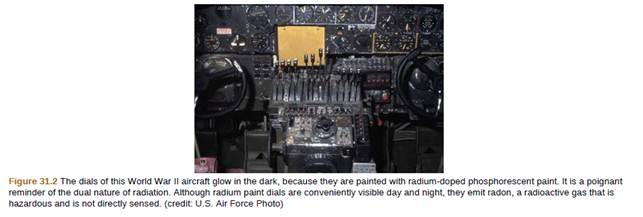Chapter 31, Problem 59PE

Chapter
Section
Textbook Problem

Data from the appendices and the periodic table may be needed for these problems.59. World War II aircraft had instruments with glowing radium−painted dials (see Figure 31.2). The activity of one such instrument was 1.0 × 10 5 Bq when new. (a) What mass of 226Ra was present? (b) After some years, the phosphors on the dials deteriorated chemically, but the radium did not escape. What is the activity of this instrument 57.0 years after it was made?To determine

(a)

What mass of R226a was present if the activity of the below instrument was 1.0×105 Bq when new?

Explanation

Given info:

R=1.0×105Bq

Formula used:

Activity,

R=0.693Nt1/2

Calculation:

Half-life of R226a=1.6×103y

We have,

R=0.693Nt 1/21.0×105=0.693N1.6× 103×3

To determine

(b)

What is the activity of this instrument 57.0 years after it was made?

Still sussing out bartleby?

Check out a sample textbook solution.

See a sample solution

The Solution to Your Study Problems

Bartleby provides explanations to thousands of textbook problems written by our experts, many with advanced degrees!

Get Started